# Polynomials

## Polynomials of Class 10

An algebraic expression f(x) of the form f(x) = a0 + a1x + a2x2 + ......+ anxn, where a0, a1, a2.......an are real numbers and all the index of x are non-negative integers is called polynomials in x and the highest Index n in called the degree of the polynomial, if

an ≠ 0.

## DEGREE OF A POLYNOMIAL:

### Zero Degree Polynomial:

Any non-zero number is regarded as a polynomial of degree zero or zero degree polynomial. For example, f(x) = a, where a ≠ 0 is a zero degree polynomial, since we can write f(x) = a as f(x) = ax0.

### (b) Constant Polynomial:

A polynomial of degree zero is called a constant polynomial. e.g. f(x) = 7.

### (c) Linear Polynomial:

A polynomial of degree 1 is called a linear polynomial.

e.g. p(x) = 4x - 3 and f(t) =  √3t + 5 are linear polynomials.

A polynomial of degree 2 is called quadratic polynomial.

e.g. f(x) = 2x2 + 5x –3/5  and g(y) = 3y2 – 5 are quadratic polynomials with real coefficients.

### VALUE OF A POLYNOMIAL:

If f(x) is a polynomial and α is any real number, then the real number obtained by replacing x by α in f(x) is called the value of f(x) at x = α and is denoted by f(α).

e.g. Value of p(x) = 5x2 - 3x + 7at x = 1 will be

∴ p(1) = 5(1)2 - 3(1) + 7

= 5 - 3 + 7 = 9

### ZEROS OF A POLYNOMIAL:

A real number α is a zero of polynomial f(x) if f(α) = 0.

The zero of a linear polynomial ax + b is -b/a . i.e.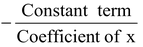Geometrically zero of a polynomial is the point where the graph of the function cuts or touches x-axis.

When the graph of the polynomial does not meet the x-axis at all, the polynomial has no real zero.

### GRAPH OF POLYNOMIALS:

In algebraic or in set theoretic language the graph of a polynomial f(x) is the collection (or set) of all points (x, y), where y = f(x). In geometrical or in graphical language the graph of a polynomial f(x) is a smooth free hand curve passing through points x1, y1), (x2, y2), (x3, y3), ..... etc. where y1, y2, y3,.... are the values of the polynomial f(x) at x1, x2, x3,.... respectively.

In order to draw the graph of a polynomial f(x), follow the following algorithm.

### ALGORITHM:

Step I: Find the values y1, y2, ...... yn of polynomial f(x) on different points x1, x2, ....... xn………… and prepare a table that gives values of y or  f(x) for various values of x.

 x: x1 x2 … xn xn+1 … y = f(x) y1 = f(x1) y2 = f(x2) … Yn = f(xn) yn+1 = f(xn+1) …

Step II: Plot that points (x1, y1), (x2, y2), (x3, y3),.....(xn, yn).... on rectangular co-ordinate system. In plotting these points use different scales on the X and Y axes.

Step III: Draw a free hand smooth curve passing through points plotted in step 2 to get the graph of the polynomial f(x).

### Graph of a Linear Polynomial:

Consider a linear polynomial f(x) = ax + b, a ≠ 0 Graph of y = ax + b is a straight line. That in why f(x) = ax + b) is called a linear polynomial. Since two points determine a straight line, so only two points need to plotted to draw the line y = ax + b. The line represented by y = ax + b crosses the X-axis at exactly one point, namely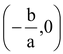.

1. Draw the graph of the polynomial f(x) = 2x - 5. Also, find the coordinates of the point where it crosses X-axis.

Sol. Let y = 2x - 5.

The following table list the values of y corresponding to different values of  x.

 x 1 4 y -3 3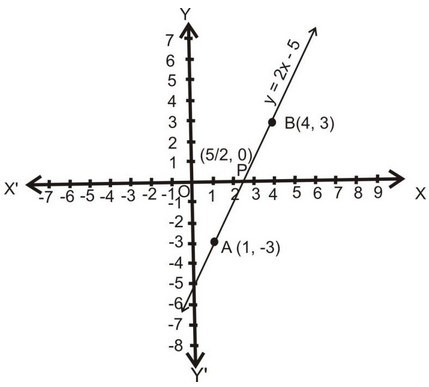The points A (1, - 3) and B (4, 3) are plotted on the graph paper on a suitable scale. A line is drawn passing through these points to obtain the graphs of the given polynomial.

### (b) Graph of a Quadratic Polynomial :

Let a,b,c be real numbers and a ≠ 0. Then f(x) = ax2 + bx + c is known as a quadratic polynomial in x. Graph of the quadratic polynomial i.e. he curve whose equation is y = ax2 + bx + c, a ≠ 0 Graph of a quadratic polynomial is always a parabola.

Let y = ax2 + bx + c, where a ≠ 0

⇒ 4ay = 4a2x2 + 4abx + 4ac

⇒ 4ay = 4a2x2 + 4abx + b2 – b2 + 4ac

⇒ 4ay = (2ax + b)2 – (b2 - 4ac)

⇒ 4ay + (b2 – 4ac) = (2ax + b)2 ⇒ 4ay + (b2 – 4ac) = 4a2 (x + b/2a)2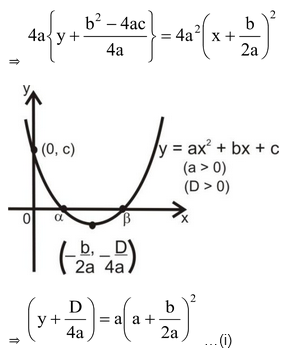where D = b2 - 4ac is the discriminate of the quadratic equation.

Remarks:

Shifting the origin at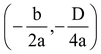, we have X = x -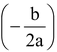and Y = y -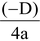Substituting these values in (i), we obtain

Y = aX2 ....(ii)

which is the standard equation of parabola

Clearly, this is the equation of a parabola having its vertex at.

The parabola opens upwards or downwards according as a > 0 or a < 0.

### SIGNS OF COEFFICIENTS OF A QUADRATIC POLYNOMIAL:

The graphs of y = ax2 + bx + c are given in figure. Identify the signs of a, b and c in each of the following: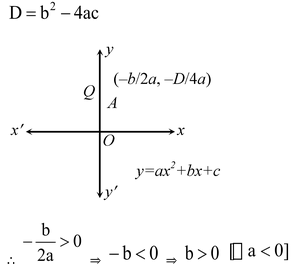(i) We observe that y = ax2 + bx + c represents a parabola opening downwards. Therefore, a < 0. We observe that the turning pointof the parabola is in first quadrant where

Parabola y = ax2 + bx + c cuts y-axis at Q. On y-axis, we have x = 0.

Putting x = 0 in y = ax2 + bx + c we get y = c.

So, the coordinates of Q are (0, c). As Q lies on the positive direction of y-axis. Therefore, c > 0.

Hence, a < 0, b > 0 and c > 0.

(ii) We find that y = ax2 + bx + c represents a parabola opening upwards. Therefore, a > 0 The turning point of the parabola is in fourth quadrant.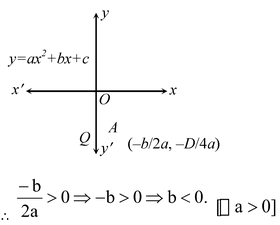Parabola y = ax2 + bx + c cuts y-axis at Q and y-axis. We have x = 0. Therefore, on putting x = 0 in y = ax2 + bx + c we get y = c.

So, the coordinates of Q are (0, c). As Q lies on negative y-axis. Therefore, c < 0.

Hence, a > 0, b < 0 and c < 0.

(iii) Clearly, y = ax2 + bx + c represents a parabola opening upwards.

Therefore, a > 0. The turning point of the parabola lies on positive direction of x-axis.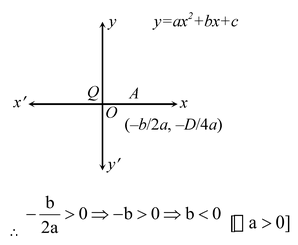The parabola y = ax2 + bx + c cuts y-axis at Q which lies on positive y-axis. Putting
x = 0 in y = ax2 + bx + c we get y = c. So, the coordinates of Q are (0, c). Clearly, Q lies on OY.

∴ c > 0.

Hence, a > 0, b < 0, and c > 0.

(iv) The parabola y = ax2 + bx + c opens downwards. Therefore, a < 0.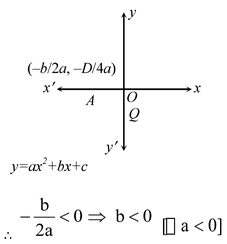The turning pointof the parabola is on negative x-axis,

Parabola y = ax2 + bx + c cuts y-axis at Q (0, c) which lies on negative y-axis. Therefore, c < 0.

Hence,  a < 0, b < 0and c < 0.

(v) We notice that the parabola y = ax2 + bx + c opens upwards. Therefore, a > 0.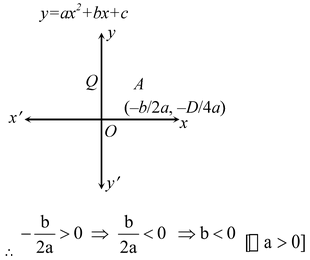Turning pointof the parabola lies in the first quadrant.

As Q (0, c) lies on positive y-axis. Therefore, c > 0.

Hence, a >0, b < 0 and c > 0.

(vi) Clearly, a < 0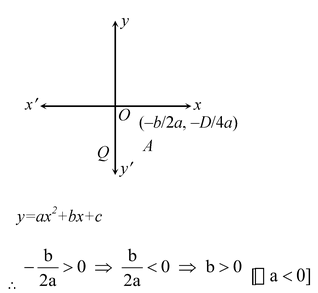Turning pointof the parabola lies in the fourth quadrant.

As Q (0, c) lies on negative y-axis. Therefore, c < 0.

Hence,  a < 0, b> 0 and c < 0.